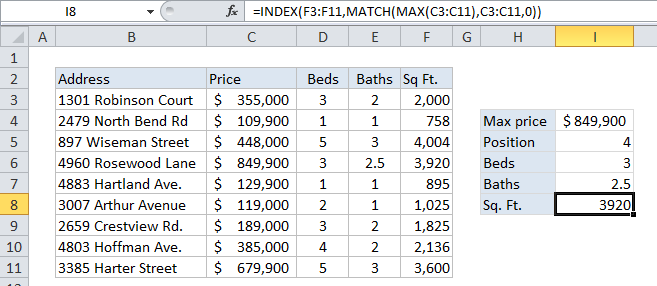## Excel Office

Excel How Tos, Tutorials, Tips & Tricks, Shortcuts

# Get information corresponding to max value in Excel

This tutorial shows how to Get information corresponding to max value in Excel using the example below;

## Formula

`=INDEX(range1,MATCH(MAX(range2),range2,0)`## Explanation

To lookup information related to the maximum value in a range, you can use a formula that combines the MAX, INDEX, and MATCH functions. In the example shown, the formula in I8 is:

`=INDEX(F3:F11,MATCH(MAX(C3:C11),C3:C11,0))`

Which returns the number 3920, representing the square footage (Sq. Ft.) of the most expensive property in the list.

Worked Example:   Get column name from index in Excel Table

### How this formula works

The MAX function first extracts the maximum value from the range C3:C11.

In this case, that value is 849900.

This number is then supplied to the MATCH function as the lookup value. The lookup_array is the same range C3:C11, and the match_type is set to “exact” with 0.

Worked Example:   Get cell content at given row and column in Excel

Based on this information, the MATCH function locates and returns the relative position of the max value in the range, which is 4 in this case.

The number 4 is supplied to INDEX as “row number” along with the range F3:F11, supplied as the lookup array. INDEX then returns the value in the 4th position in F3:F11m which is 3924.

Worked Example:   Vlookup Examples in Excel

Note: in case of duplicates (i.e. two or more max values that are the same) this formula will return info for the first match, the default behavior of the MATCH function.